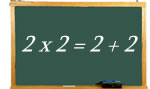Logic puzzles, riddles, math puzzles and brainteasers - pzzls.com

Vandaag is het 10 August 2022

# 2 x 2 = 2 + 2 - math puzzle

Difficulty:Rating: 2.9/5.0

 Two times two is equal to two and two. But are there more number pairs for which the sum of the numbers is is equal to the product of the numbers? The numbers may be different.